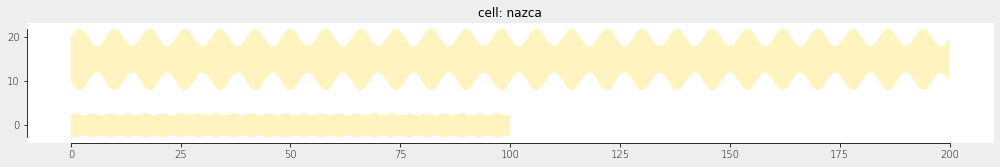Home Forums Nazca Questions and Answers Drawing line with mathematical function Reply To: Drawing line with mathematical function

#6049

Dear Cameron,

If I interpret your question correctly, i.e. that you want to modulate the edge of the waveguide, there are a few ways to get a sin-shaped waveguide edge.

The most flexible is to define a parametric curve in x(t), y(t), and w(t), where in your case you exploit w(t). See also Free form curves, which discusses arbitrary parametric curves.

``````import numpy as np
import nazca as nd

xs.width = 5.0

# create functions x, y, w for the parametric waveguide:
def x(t, wavelength, periods, **kwargs):
"""X as function of t and free parameters."""
return periods * wavelength * t
def y(t, **kwargs):
"""Y as function of t and free parameters."""
return 0
def w(t, width1=None, width2=None, amplitude=1.0, periods=10, **kwargs):
"""Width as function of t, width1 and width2 and free parameters."""
return width1 + amplitude * np.sin(t * periods * np.pi)

# create the new parametric function using the template Tp_viper():
params = {'wavelength': 2.0, 'amplitude': 0.5, 'periods': 50}  # assign *all* free params used in functions x, y, and w.
sin_edge = nd.Tp_viper(x, y, w, xs='myXS', **params)

# put waveguides:
sin_edge().put(0)
sin_edge(width1=10.0, wavelength=4.0, amplitude=4.0, N=2000).put(0, 15)
nd.export_gds()``````

The result is the following waveguides:The second solution is to define a perturbation of the edge of a straight waveguide element. This hasn’t been an actively in use option, but I dusted it off for nazca-0.5.10 to work in the following way (not using a sin but 2*t for simplicity). The edge1 function in t adds to the normal width of the guide, but it becomes absolute when using width=1.0.

``````import nazca as nd
s = nd.strt(length=10, edge1=lambda t: 2*t + 1.0, edgepoints=100, layer=1).put(0)
nd.export_gds()``````

The above would work the same for straight interconnects.

Lastly, you can draw your desired waveguide directly as a polygon:

``````import nazca as nd
points = [(x1, y1), (x2, y2), ...] # your shape
nd.Polygon(points=points, layer=1).put(0)
nd.export_gds()``````

You ideally would put this in a cell and add pins to it as described in Make and put a Cell.

Ronald